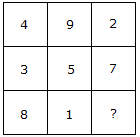# Verbal Reasoning - Character Puzzles - Discussion

### Discussion :: Character Puzzles - Character Puzzles 1 (Q.No.27)

27.

Which one will replace the question mark ?[A]. 9 [B]. 6 [C]. 15 [D]. 14

Explanation:

(4 + 9 + 2) = (3 + 5 + 7) = (8 + 1 + 6)

Total in each case = 15.